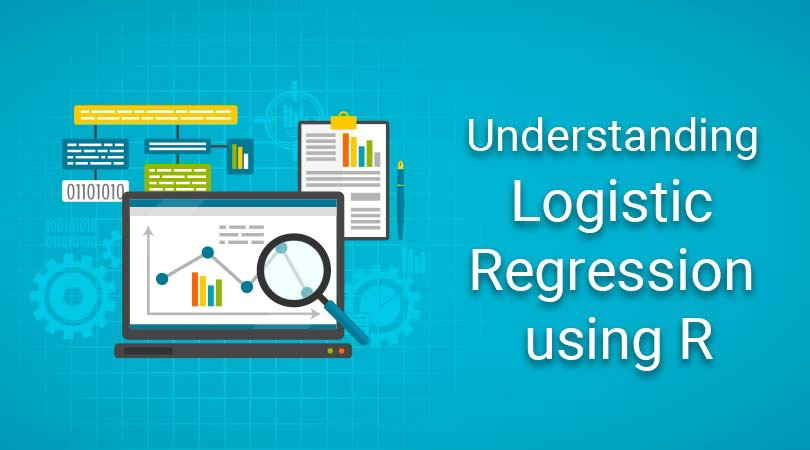# Understanding Logistic Regression Using R | ExcelR## Why Is Logistic Regression the Spokesperson of Binomial Regression Models?

Why Is Logistic Regression the Spokesperson of Binomial Regression Models? A small discussion on the binomial regression model and its link functions

## Regression Algorithm Part 1: Linear Regression Using R Language

What is a Linear Regression? Linear Regression is one of the supervised machine learning algorithms to predict values within a continuous range.

## 7 steps to run a linear regression analysis using R

7 steps to run a linear regression analysis using R. I learned how to do regression analysis in R using brute force. With these 7 copy and paste steps, you can too.

## Logistic Regression : Intuition of Logistic Regression

Prerequisite: This assumed that you understand the concept of supervised algorithm and preliminary difference between classification and regression. Otherwise you can refer here.

## Regression: Linear Regression

Machine learning algorithms are not your regular algorithms that we may be used to because they are often described by a combination of some complex statistics and mathematics.